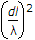# Electronics and Communication Engineering - Electromagnetic Field Theory - Discussion

5.

A broadside array operating at 100 cm wavelength consist of 4 half-wave dipoles spaced 50 cm apart. Each element carries radio frequency current in the same phase and of magnitude 0.5 A. The radiated power will be

 [A]. 196 W [B]. 73 W [C]. 36.5 W [D]. 18.25 W

Explanation:

P = n . I2 . Rr, where Rr = 80p2Ω.

n = 4.

 Pratik said: (Aug 26, 2014) What will be "dl" here?

 Alex said: (Apr 10, 2015) Could you please put the complete equation?

 Usha said: (Oct 10, 2015) There dl=50.

 Blessy said: (Sep 19, 2016) What is the value of p in the Rr equation?

 Vivek said: (Nov 9, 2016) n = 4; i = 0.5; Here p is pi(3.14....);dl = 50cm. P = n * I^(2) * Rr = >;P = Rr; Rr = 80 * (9.8) * (1/4) = 196 W;(pi^(2) approximately 9.8).

 Pranab said: (Mar 4, 2017) But half dipole Rr=73 ohm.

 Chandraraj said: (Jul 18, 2018) The correct one is option C.

 Krishna Chaitanya said: (Feb 24, 2020) P= n I^2 Rr. Where n=4, I=0.5=1/2A, Rr=73 ohms. So P= (4) (1/2) ^2 (73) = 73W. CORRECT OPTION IS B.